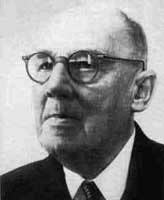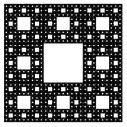A# Sierpinski, Waclaw Franciszek (1882–1969)Sierpinski gasket.

Waclaw Sierpinski was a Polish mathematician who made outstanding contributions to set theory, which included research on the axiom of choice and the continuum hypothesis, number theory, and topology. Two well-known fractals, the Sierpinski carpet and the Sierpinski gasket (see below), are named after him.## Sierpinski carpet

The Sierpinski Carpet is a fractal, named after Waclaw Sierpinski, that is derived from a square by cutting it into nine equal squares with a 3 × 3 grid, removing the central piece, and then applying the same procedure ad infinitum to the remaining eight squares. It is one of two generalizations of the Cantor set to two dimensions; the other is the Cantor dust. The Carpet's Hausdorff dimension is log 8/log 3 = 1.8928...## Sierpinski gasket

The Sierpinski gasket is a fractal, also known as the Sierpinski Triangle or Sierpinski sieve, produced by the following set of rules:

1. Start with any triangle in a plane.
2. Shrink the triangle by ½, make three copies, and translate them so that each triangle touches the two other triangles at a corner.
3. Repeat step 2 ad infinitum.

The gasket can also be made by starting with Pascal's triangle, then coloring the even numbers white and the odd numbers black. Most curiously, it can be generated by a game of chance. Start with three points, labeled 1, 2, and 3, and any starting point, S. Then select randomly 1, 2, or 3, using a die or some other method. Each random number defines a new point halfway between the latest point and the labeled point that the random number indicates. When the game has gone on long enough the pattern produced is the Sierpinski gasket.

The gasket has a Hausdorff dimension of log 3/log 2 = 1.585..., which follows from the fact that it is a union of three copies of itself, each scaled by a factor of ½. Adding rounded corners to the defining curve gives a non-intersecting curve that traverses the gasket from one corner to another and which Benoit Mandelbrot called the Sierpinski arrowhead.